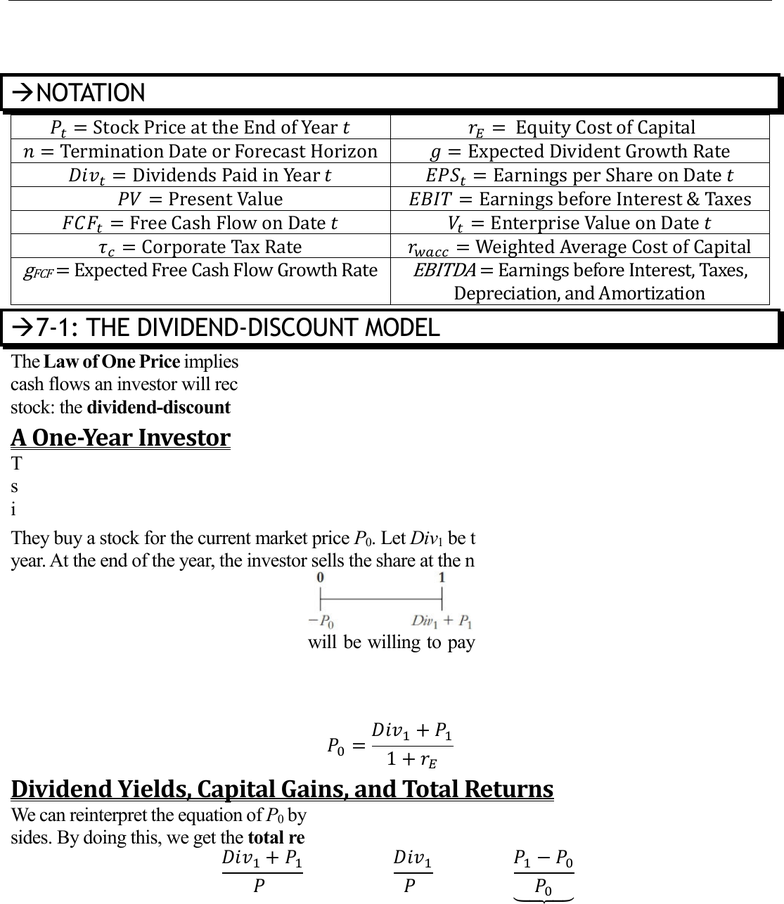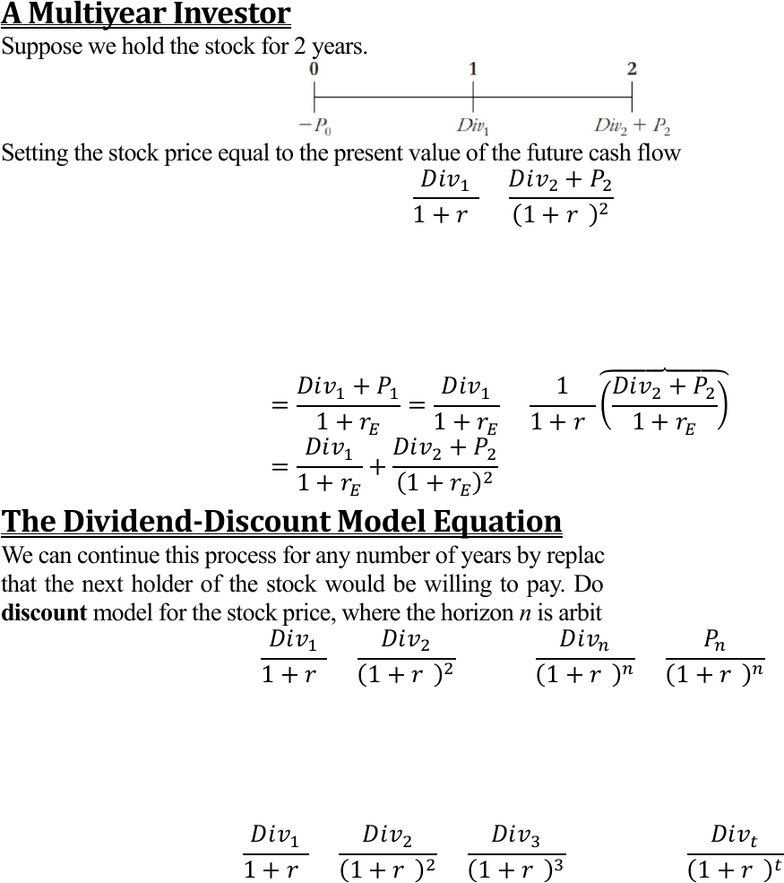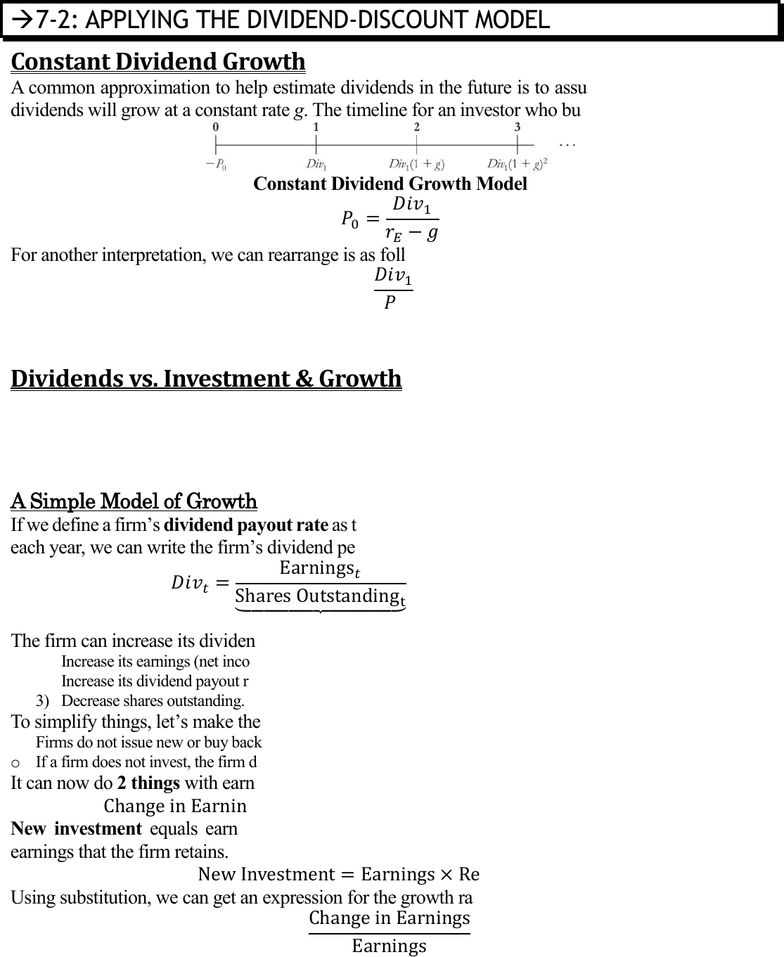Textbook Notes (290,000)
CA (170,000)
UTM (8,000)
Chapter 7

# MGT338H5 Chapter Notes - Chapter 7: Dividend Discount Model, Dividend Yield, Cash Flow

Department
Management
Course Code
MGT338H5
Professor
Gabor Virag
Chapter
7

This preview shows pages 1-3. to view the full 11 pages of the document.Prof. Gabor Virag MGT338 Page 1 of 11
Chapter 7 Valuing Stocks
Chapter 7 Valuing Stocks
Dividend-Discount Model; Total Payout & Free Cash Flow Valuation Models; Valuation
Based on Comparable Firms; Information, Competition, and Stock Prices
NOTATION




 
 
 
 
 


 
7-1: THE DIVIDEND-DISCOUNT MODEL
The Law of One Price implies that the price of a security should equal the present value of the expected
cash flows an investor will receive from owning it. Using this, we derive the first method of valuing a
stock: the dividend-discount model.
A One-Year Investor
There are 2 potential sources of cash flows from owning a stock; dividends and selling the share at
some future date. The total amount received in dividends and from selling the stock will depend on the
investors investment horizon. Lets consider a 1-year investor.
They buy a stock for the current market price P0. Let Div1 be the total dividends paid at the end of the
year. At the end of the year, the investor sells the share at the new market price P1.
Given these expectations, the investor will be willing to pay a price today up to the point that this
transaction has a zero NPV. Since there’s some risk involved, we must discount these cash flows based
on the equity cost of capital rE. This is the expected return of other investments available in the market
with equivalent risk to the firm’s shares.

Dividend Yields, Capital Gains, and Total Returns
We can reinterpret the equation of P0 by multiplying by (1 + rE), divide by P0, and subtract 1 from both
sides. By doing this, we get the total return.

  


The dividend yield is the expected annual dividend of the stock divided by its current price. Its the
percentage return the investor expects to earn from the dividend paid by the stock.

Only pages 1-3 are available for preview. Some parts have been intentionally blurred.Prof. Gabor Virag MGT338 Page 2 of 11
Chapter 7 Valuing Stocks
The capital gain is what the investor will earn on the stock, P1 P0. We divide it by the current stock
price to express the capital gain as a percentage return, called the capital gain rate.
The sum of the dividend yield and capital gain rate is called the total return, which is what an investor
will earn for a 1-year investment. The equation states that the expected total return of the stock should
equal the expected return of other investments available in the market with equivalent risk.
If a stock offers a higher return than other securities with the same risk, investors would buy the stock
and put upward pressure on the price. This increase in the price would lower the dividend yield and
capital gain rate. The opposite is also true; lower return causes price to decrease, which increases total
return until the equation is satisfied.
A Multiyear Investor
Suppose we hold the stock for 2 years.
Setting the stock price equal to the present value of the future cash flows in this case implies:


Note: There is no underlying difference between the 1-year equation and the 2-year equation. In the 1-
year equation, there is no second term and the selling price of the stock is in the numerator of the first
term as P1. However, P1 is determined by the expected future cash flows. In other words, we can get
the second equation from the first one:





The Dividend-Discount Model Equation
We can continue this process for any number of years by replacing the final stock price with the value
that the next holder of the stock would be willing to pay. Doing so leads to the general dividend-
discount model for the stock price, where the horizon n is arbitrary:



This applies to a single n-year investor, who collects dividends for n years and then sells the stock, OR
applies to a series of investors who hold the stock for shorter periods and then resell it.
For the special case in which the firm eventually pays dividends and is never acquired, it is possible to
hold the shares forever. Consequently, we can let n go to infinity:



  

That is, the price of the stock is equal to the present value of the expected future dividends it will pay.

Only pages 1-3 are available for preview. Some parts have been intentionally blurred.Prof. Gabor Virag MGT338 Page 3 of 11
Chapter 7 Valuing Stocks
7-2: APPLYING THE DIVIDEND-DISCOUNT MODEL
Constant Dividend Growth
A common approximation to help estimate dividends in the future is to assume that, in the long run,
dividends will grow at a constant rate g. The timeline for an investor who buys and holds a stock is:
Constant Dividend Growth Model

For another interpretation, we can rearrange is as follows:

We see that g equals the expected capital gain rate. In other words, with constant expected dividend
growth, the expected growth rate of the share price matches the growth rate of dividends.
Dividends vs. Investment & Growth
With the equation above, we now know that a firm’s share price increases with the current dividend
level Div1 and the expected growth rate g. To maximize share price, a firm wants to increase both.
However, there’s a tradeoff; increasing growth may require investment but money spent on investment
cannot be used to pay dividends.
A Simple Model of Growth
If we define a firms dividend payout rate as the fraction of its earnings that the firm pays as dividends
each year, we can write the firms dividend per share at date t as follows:




The firm can increase its dividends in 3 ways:
1) Increase its earnings (net income).
2) Increase its dividend payout ratio.
3) Decrease shares outstanding.
To simplify things, lets make the following assumptions:
o Firms do not issue new or buy back shares. The number of shares outstanding is fixed.
o If a firm does not invest, the firm does not grow, so earnings generated remains constant.
It can now do 2 things with earnings; pay dividends, or it retain/reinvest them. Therefore:
  
New investment equals earnings multiplied by the firms retention rate, the fraction of current
earnings that the firm retains.
 
Using substitution, we can get an expression for the growth rate of earnings:
  

 
If the firm chooses to keep its dividend payout rate constant, then the growth in dividends will equal
growth of earnings:   